In frozen core dams, the impermeable elements are frozen to maintain a water-tight region in the dam throughout the operational life of the structure. Such dams are common in the Subarctic and Arctic climate zones due to naturally occurring ground freezing mechanisms and the fact that low permeability core materials are not available or not suitable.

Proper frozen dam design is impossible without thermal analyses of the constructions, the thermophysical properties of the soil, and the meteorological and hydrological conditions.

Frost 3D is the most effective tool to perform such simulations, with its ability to predict soil freezing and thawing with water filtration effects included.

The Frost 3D software package was used to compute water filtration through the dam and core freezing using subsurface cooling units.

Dimensions of simulated site: length – 400 m, width – 120 m and height – 72 m (Figure 1).Figure 1: Specifying thermal and hydrological conditions

The 80 cooling units, each 50 m in length, are embedded in the dam. To numerically solve the filtration equations and thermal conductivity, a 3D geometric model of the dam is discretized with a 12 336 576-point mesh (Figure 2).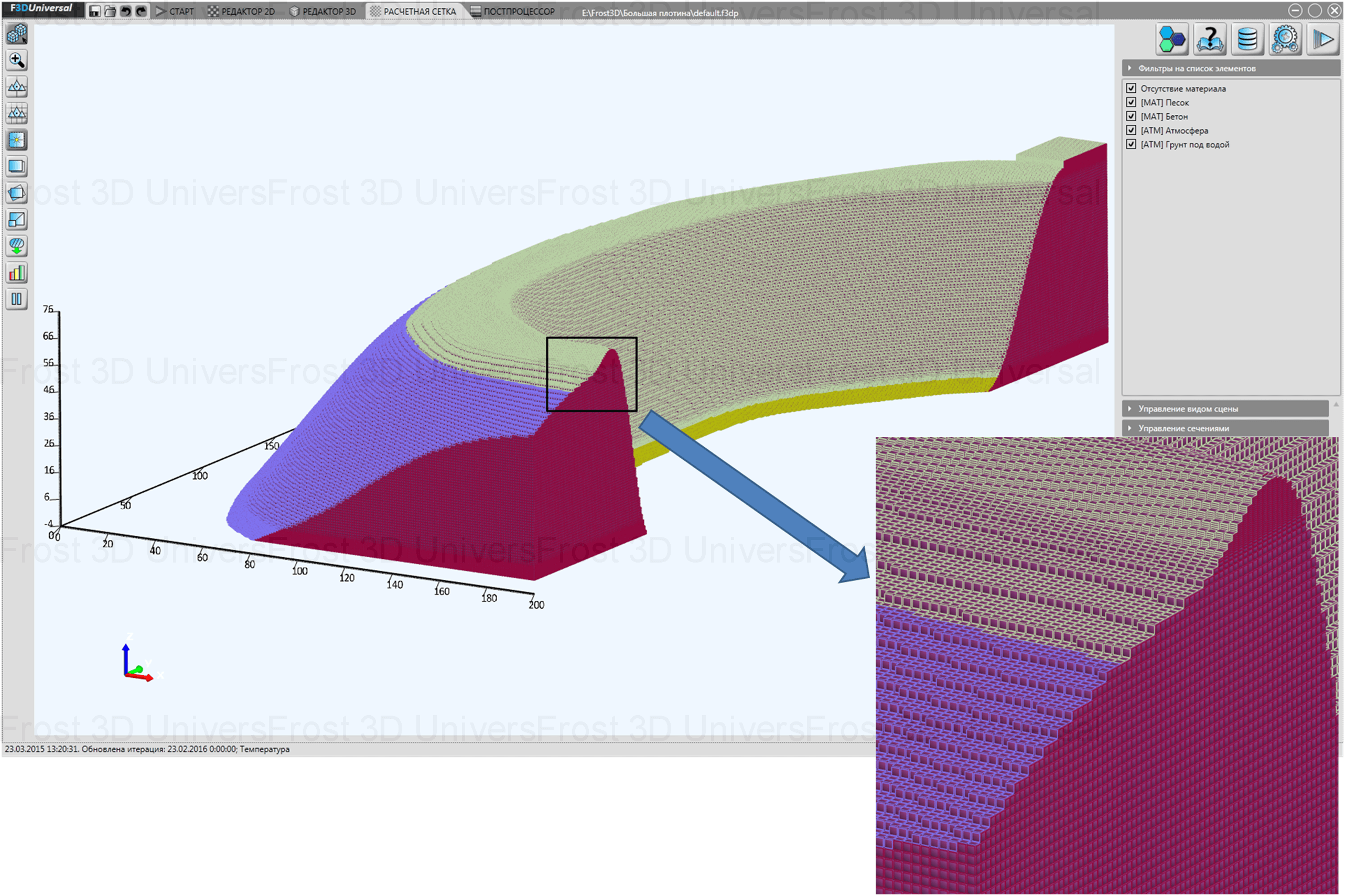Figure 2: Mesh generated by Frost 3D

At the top of the dam, we specify boundary conditions for heat transfer with the atmosphere: the air temperature dynamics and the relationship between the heat transfer coefficient and wind speed (Figure 3).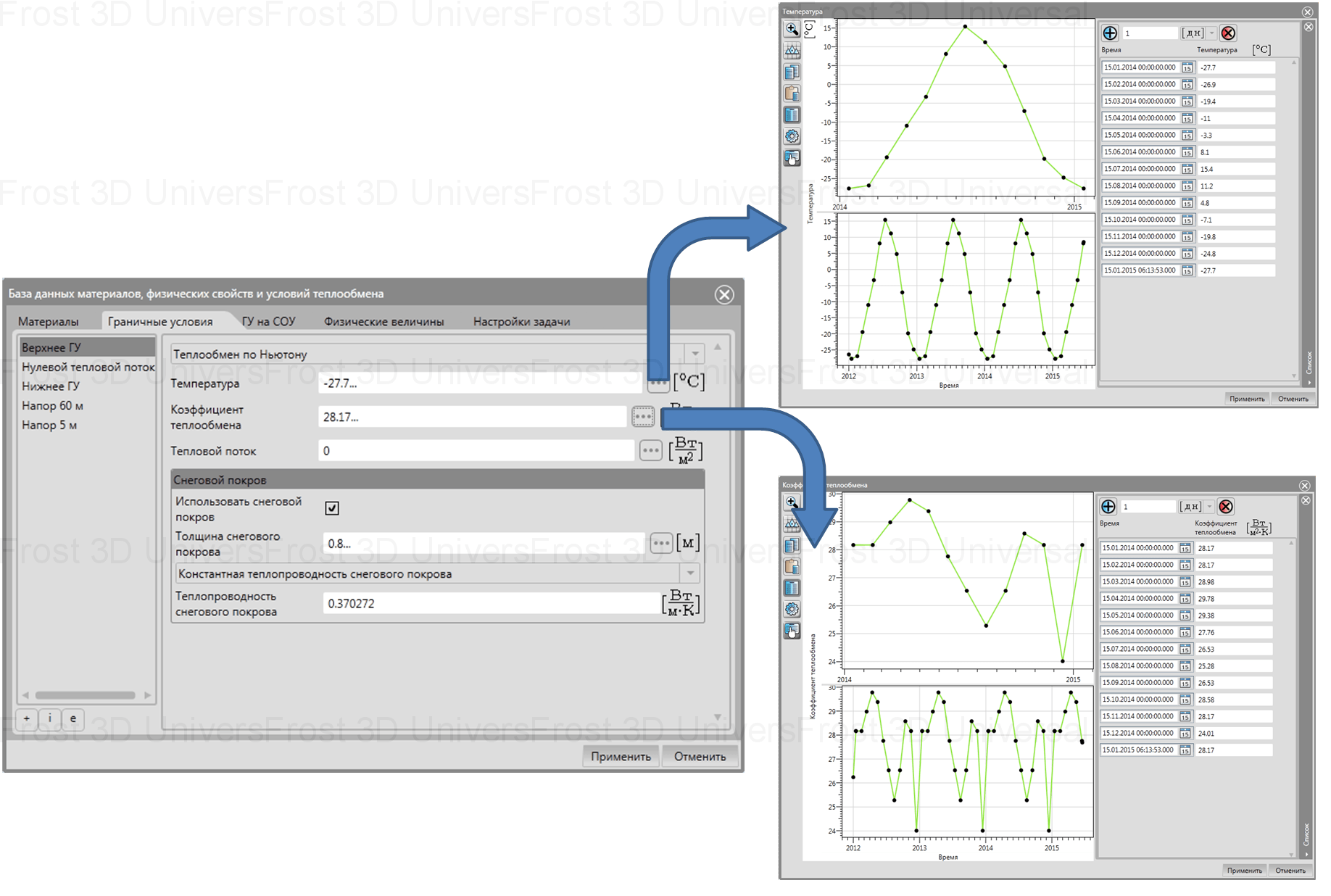Figure 3: Specifying parameters for heat transfer with the atmosphere in Frost 3D software package

For the submerged parts of the dam, we specify the heat transfer conditions for water: the water temperature dynamics and the ground-water heat transfer coefficient (Figure 4).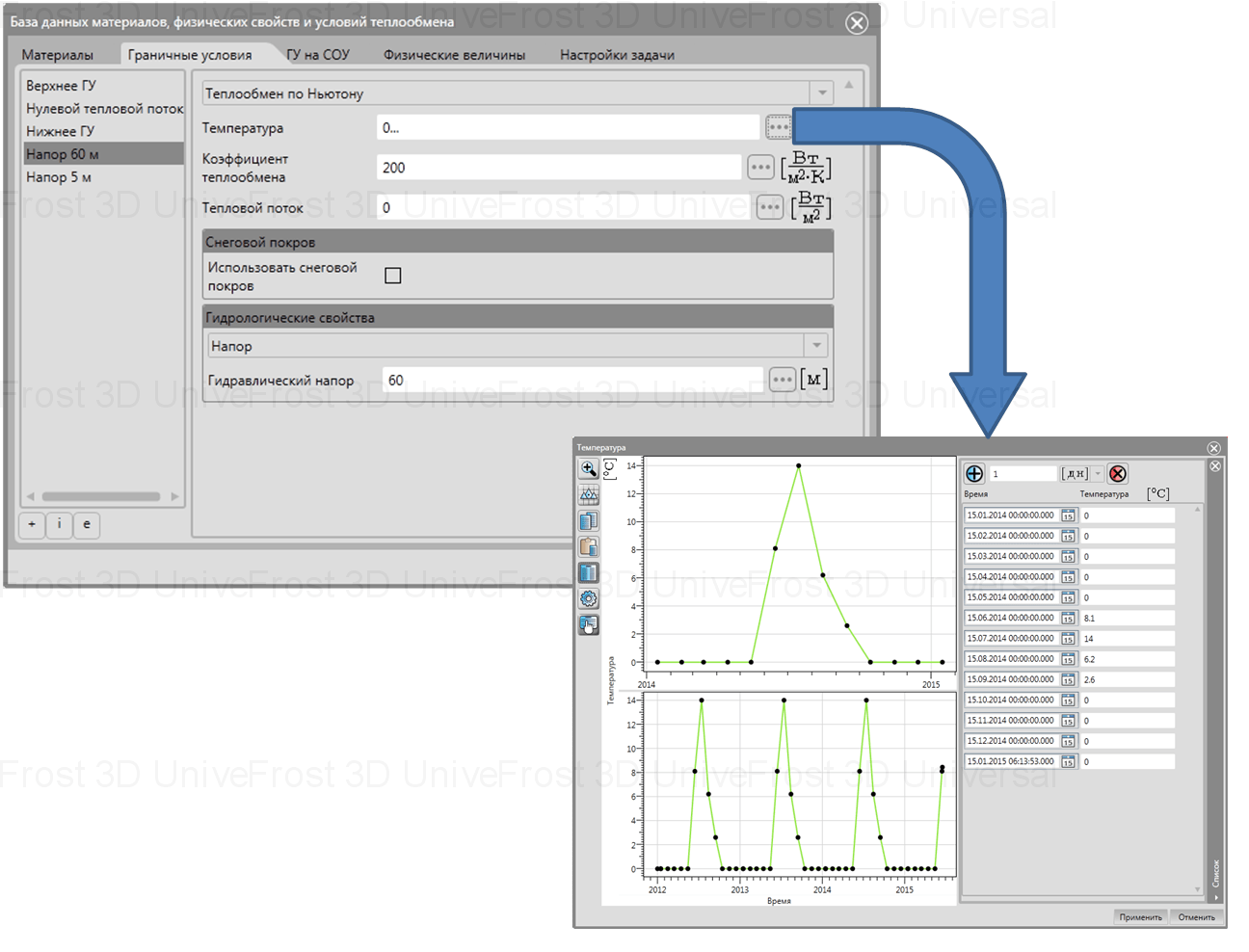Figure 4: Specifying heat transfer parameters for water in the Frost 3D software package

To simulate water filtration in these parts of the dam, hydraulic heads are set 60 m from the water reservoir and 5 m from the river. The heat flux is calculated on cooling units with respect to the wind speed and air temperature (Figure 5).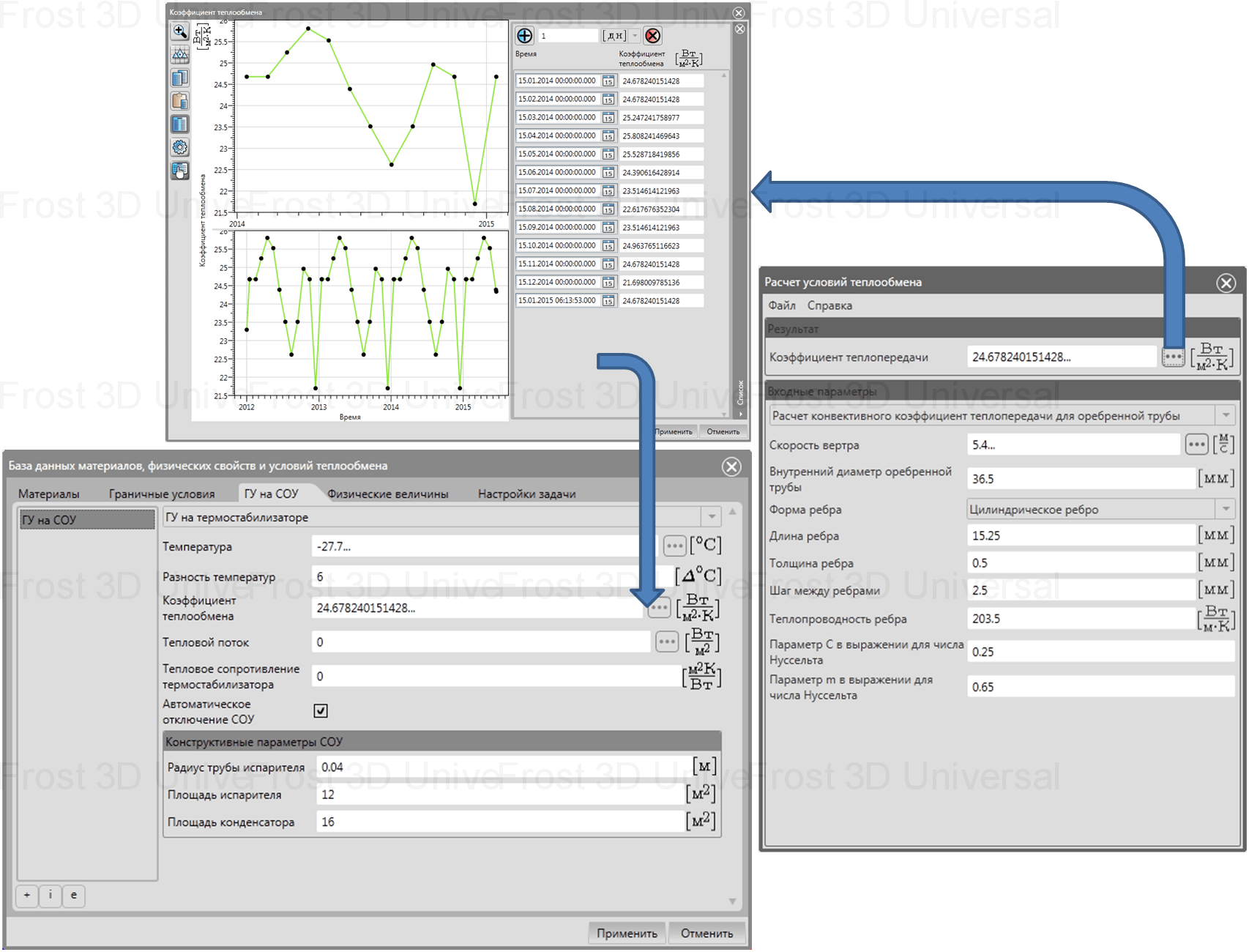Figure 5: Specifying cooling unit parameters to calculate evaporator heat flux

The thermophysical properties and the filtration coefficient are specified for the dam material (Figure 6).Figure 6: Specifying thermophisical properties of the dam in Frost 3D

With this data, the Frost 3D software package can compute the water filtration velocity and the temperature distribution in the dam for 3 years (Figures 7 to 9).Figure 7: Distribution of filtration velocity along x-axis in µm/s. Horizontal section at Z = 26 mFigure 8: Temperature distribution in the dam core in February of the first winter (computed filtration). Horizontal section at Z = 68 mFigure 9: Temperature distribution in the dam core in February of the first winter (computed filtration). Vertical section at Y = 362 m

The distribution of the water filtration velocity in the computational domain is complex (typically non-constant) (Figure 7). Accordingly, when solving for soil freezing or thawing with water filtration, it is necessary to compute water-flow rates rather than use constant velocity values. User-defined water velocities will differ from computed values and consequently distort the ground temperature simulation output. Below is the distribution of filtration velocity resulting from the initial user-defined value of 25 µm/s (Figure 10). Note that when the soil freezes, the filtration velocity approaches zero.Figure 10: Distribution of filtration velocity along x-axis in µm/s (user-defined). Horizontal section at Z = 26 m

The temperature field obtained after specifying the filtration velocity (Figure 11) differs from the temperature field resulting from the computation of filtration velocity via numerical solution of the filtration equation (Figure 8).Figure 11: Temperature distribution in the first winter of freezing of the dam core. The filtration velocity is user-defined. Horizontal section at Z = 68 m

It is more representative to visualize the temperature field in the cross-section near the cooling units (Figure 12). Without filtration velocity computation of, the inaccuracy in the determination of the freezing area around the cooling units can reach 1.5 meters.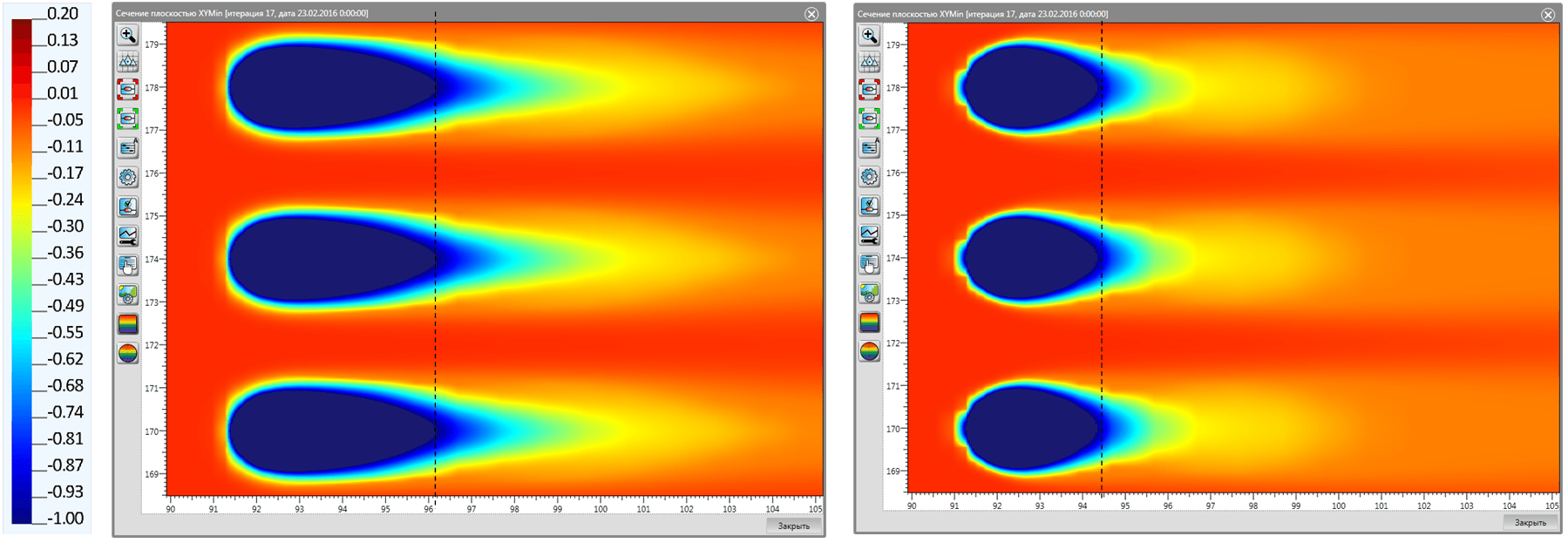a) b)

Figure 12: Temperature distribution around cooling units for computed (а) and user-defined (b) filtration velocity. Horizontal section at Z = 16 m